# Data Models in DBMS

Challenge Inside! : Find out where you stand! Try quiz, solve problems & win rewards!
Learn via video courseDBMS Course - Master the Fundamentals and Advanced Concepts
By Srikanth VarmaFree
5Enrolled: 1000DBMS Course - Master the Fundamentals and Advanced Concepts
Srikanth VarmaFree
5
Enrolled: 1000

## Overview

Nowadays, all enterprises require properly defined and formatted data to bring all the segments of an enterprise - IT, Management, and others together. Data models play a key role in solving this problem as they represent an enterprise's data and connections between them in a pictorial form.

## What are Data Models in DBMS?

Data models in DBMS help to understand the design at the conceptual, physical, and logical levels as it provides a clear picture of the data making it easier for developers to create a physical database.

Data models are used to describe how the data is stored, accessed, and updated in a DBMS. A set of symbols and text is used to represent them so that all the members of an organization can understand how the data is organized. It provides a set of conceptual tools that are vastly used to represent the description of data.

There are many types of data models that are used in the industry.

## Types of Data Models in DBMS

### Hierarchical Model

The hierarchical data model is one of the oldest data models, developed in the 1950s by IBM. In this data model, the data is organized in a hierarchical tree-like structure. This data model can be easily visualized because each record in DBMS has one parent and many children (possibly 0) as shown in the image given below.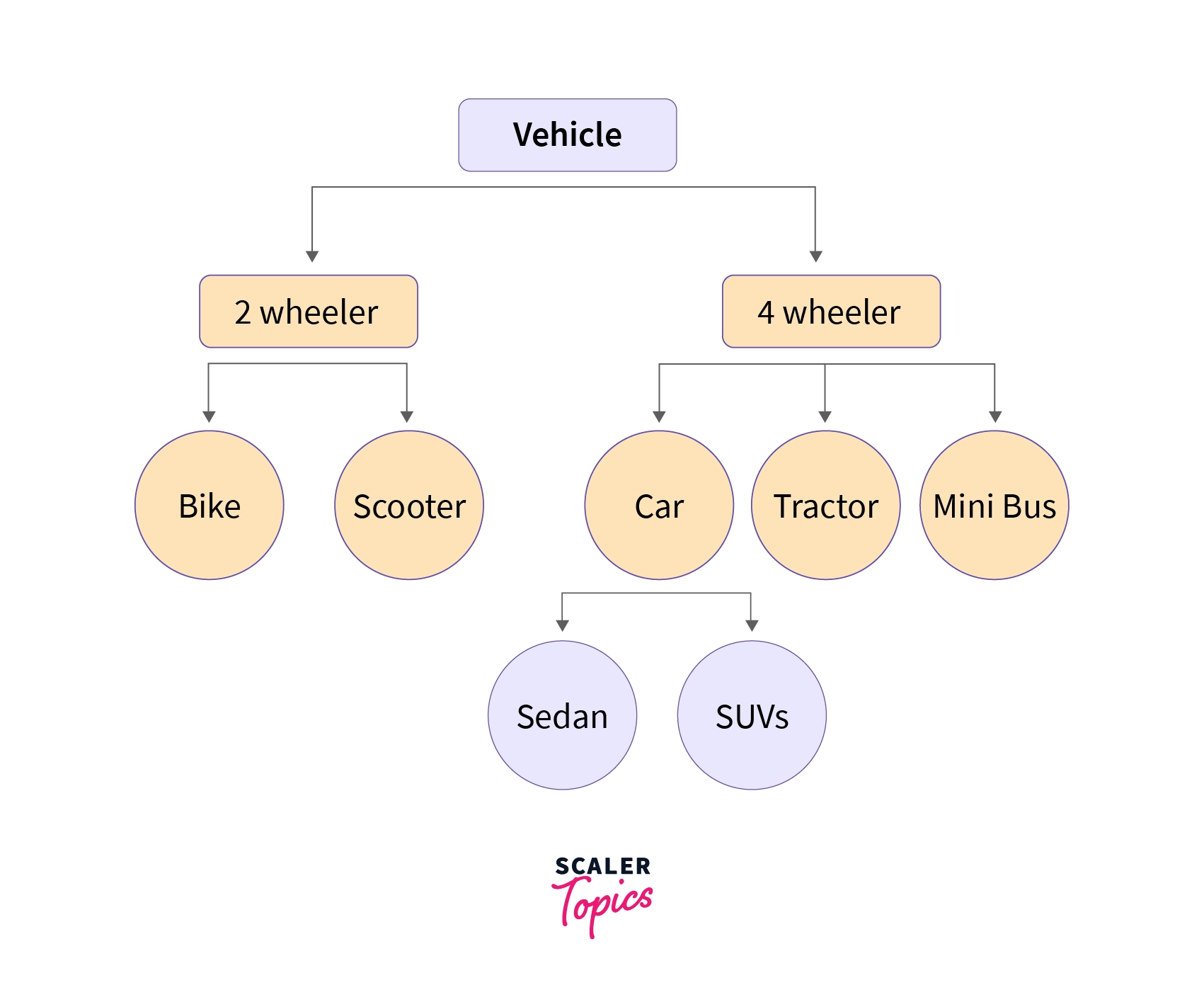The above-given image represents the data model of the Vehicle database, vehicle are classified into two types Viz. two-wheelers and four-wheelers and then they are further classified.

The main drawback we can see here is we can only have one too many relationships under this model, hence the hierarchical data model is very rarely used nowadays.

### Network Model

A network model is nothing but a generalization of the hierarchical data model as this data model allows many to many relationships therefore in this model a record can also have more than one parent.

The network model in DBMS can be represented as a graph and hence it replaces the hierarchical tree with a graph in which object types are the nodes and relationships are the edges.

For example -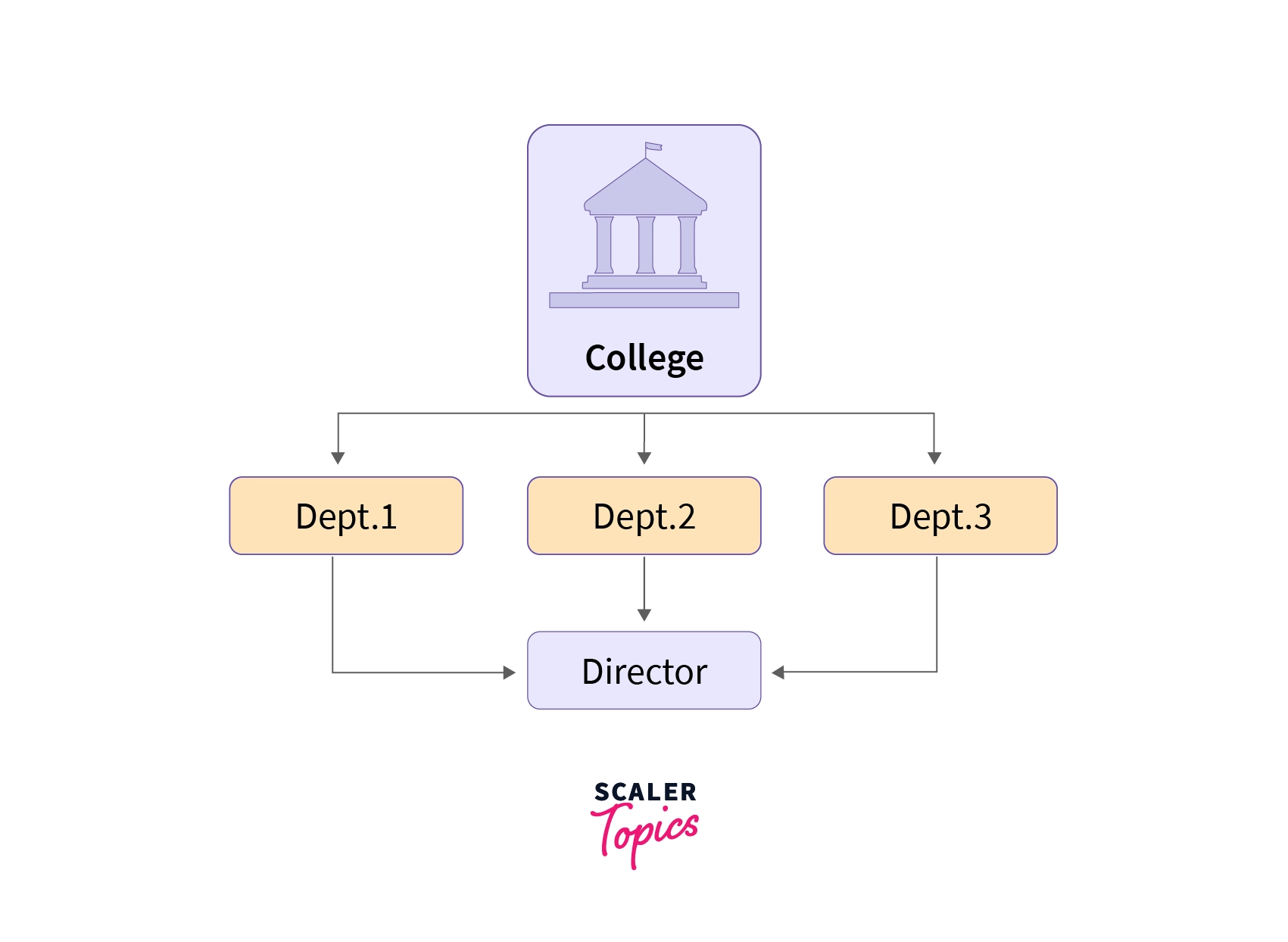Here you can see all three departments are linked with the director which was not possible in the hierarchical data model.

In the network model, there can be many possible paths to reach a node from the root node (College is the root node in the above case), therefore the data can be accessed efficiently when compared to the hierarchical data model. But, on the other hand, the process of insertion and deletion of data is quite complex.

### Entity-Relationship Model (ER Model)

An Entity-Relationship model is a high-level data model that describes the structure of the database in a pictorial form which is known as ER-diagram. In simple words, an ER diagram is used to represent logical structure of the database easily.

ER model develops a conceptual view of the data hence it can be used as a blueprint to implement the database in the future.
Developers can easily understand the system just by looking at ER diagram. Let's first have a look at the components of an ER diagram.

They are represented as rectangles in the ER diagram. For example - Car, house, employee.

• Entity Set - A set of the same type of entities is known as an entity set. For example - Set of students studying in a college.
• Attributes - Properties that define entities are called attributes. They are represented by an ellipse shape.
• Relationships - A relationship in DBMS is used to describe the association between entities. They are represented as diamond or rhombus shapes in the ER diagram.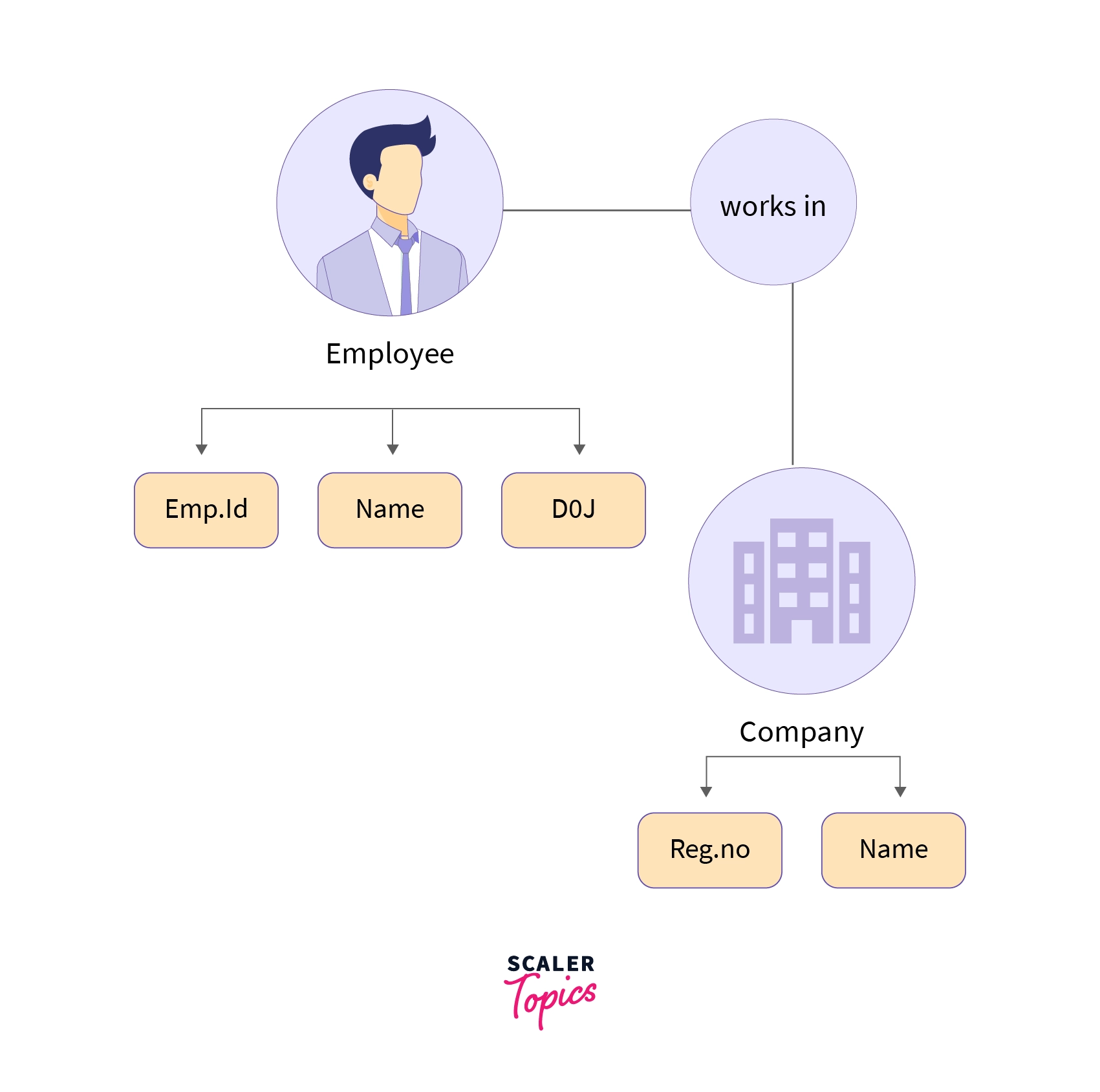In the above-represented ER diagram, we have two entities that are Employee and Company, and the relationship among them. Also, in the above-represented ER diagram, we can see that both the employee and company have some attributes and the relationship is of "works in" type, which means the employee works in a company.

### Relational Model

This is the most widely accepted data model. In this model, the database is represented as a collection of relations in the form of rows and columns of a two-dimensional table. Each row is known as a tuple (a tuple contains all the data for an individual record) while each column represents an attribute. For example -

Stu. IdNameBranch
101NamanCSE
102SaloniECE
103RishabhIT
104PulkitME

The above table shows a relation "STUDENT" with attributes such as Stu. Id, Name, and Branch which consists of 4 records or tuples.

### Object-Oriented Data model

As suggested by its name, the object-oriented data model is a combination of object-oriented programming and relational data model. In this data model, the data and their relationship are represented in a single structure which is known as an object.

Since data is stored as objects we can easily store audio, video, images, etc in the database which was very difficult and inconvenient to do in the relational model. As shown in the image below two objects are connected with each other through links.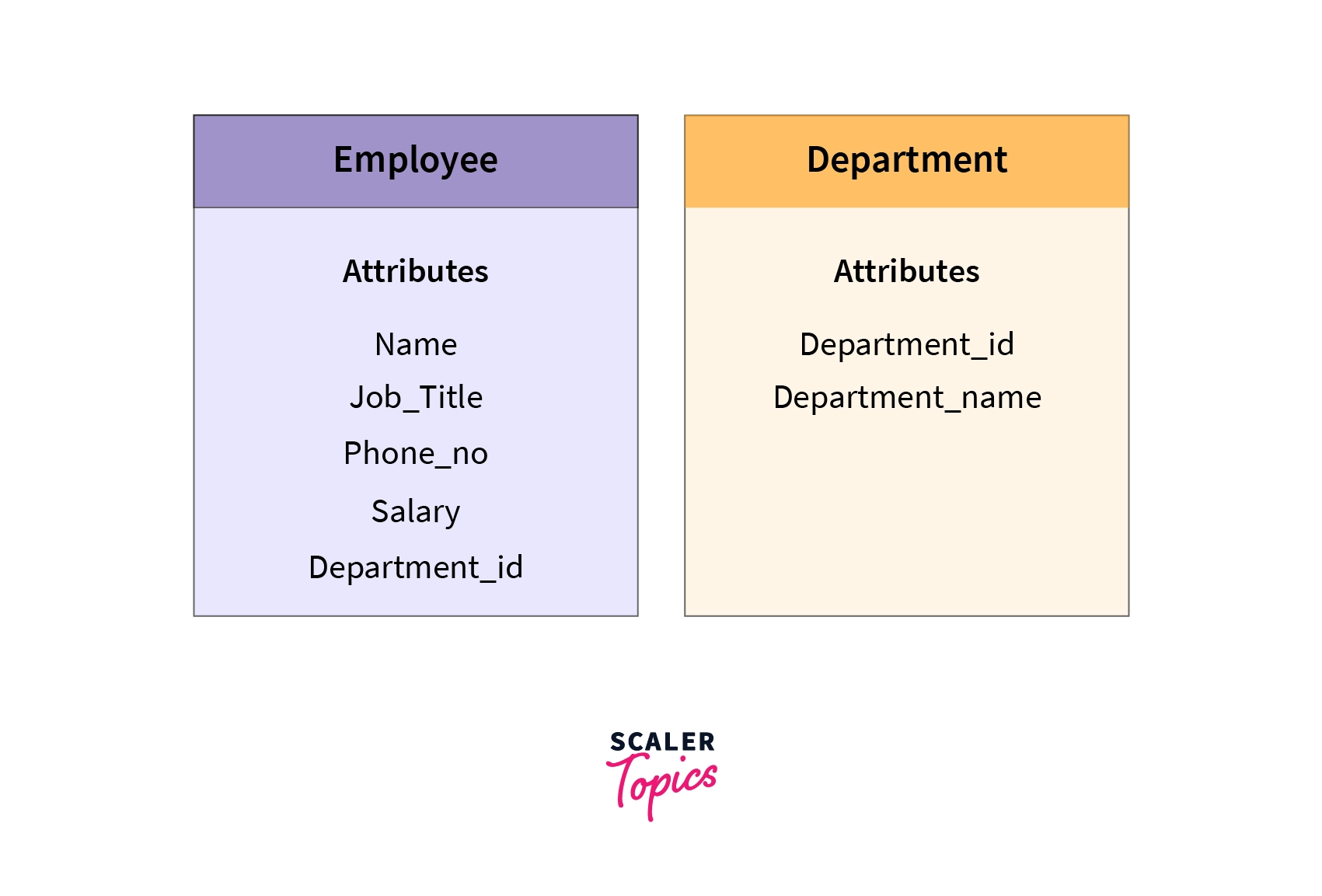In the above image, we have two objects that are Employee and Department in which all the data is contained in a single unit (object). They are linked with each other as they share a common attribute $i.e.$ Department_Id.

### Object Relational Data Model

Again as suggested by its name, the object-relational data model is an integration of the object-oriented model and the relational model. Since it inherits properties from both of the models it supports objects, classes, etc like object-oriented models, and tabular structures like the relational model.

For example -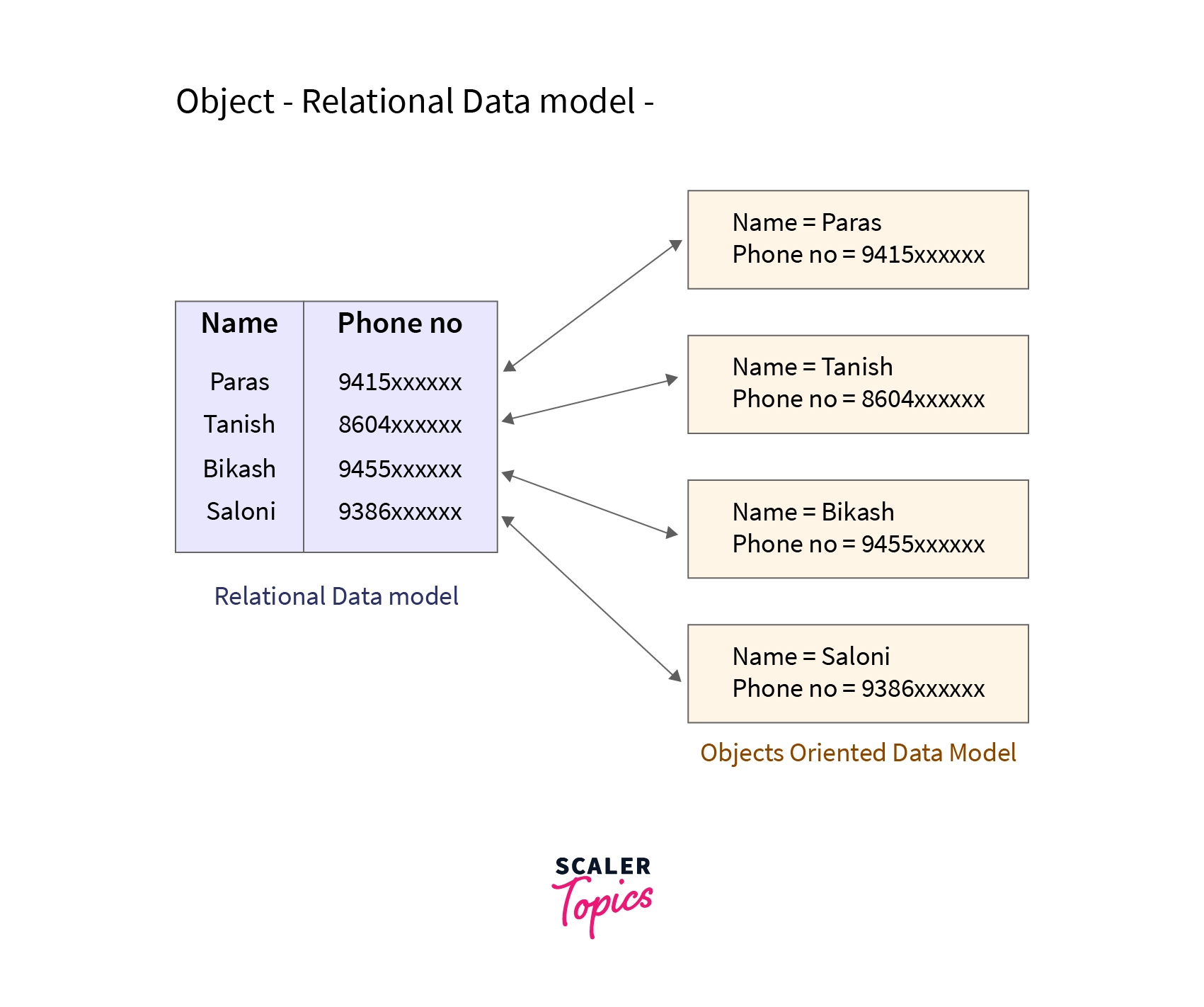It provides data structures and operations used in the relational model and also provides features of object-oriented models like classes, inheritance, etc. The only drawback of this data model is that it is complex and quite difficult to handle.

### Float Data Model

The float data model consists of a single two-dimensional array of data elements. For example, in the 2-d array we can have one column as username and the other as password. One thing that can be noticed here is, there should not be duplicate entries in the table (array).

For example -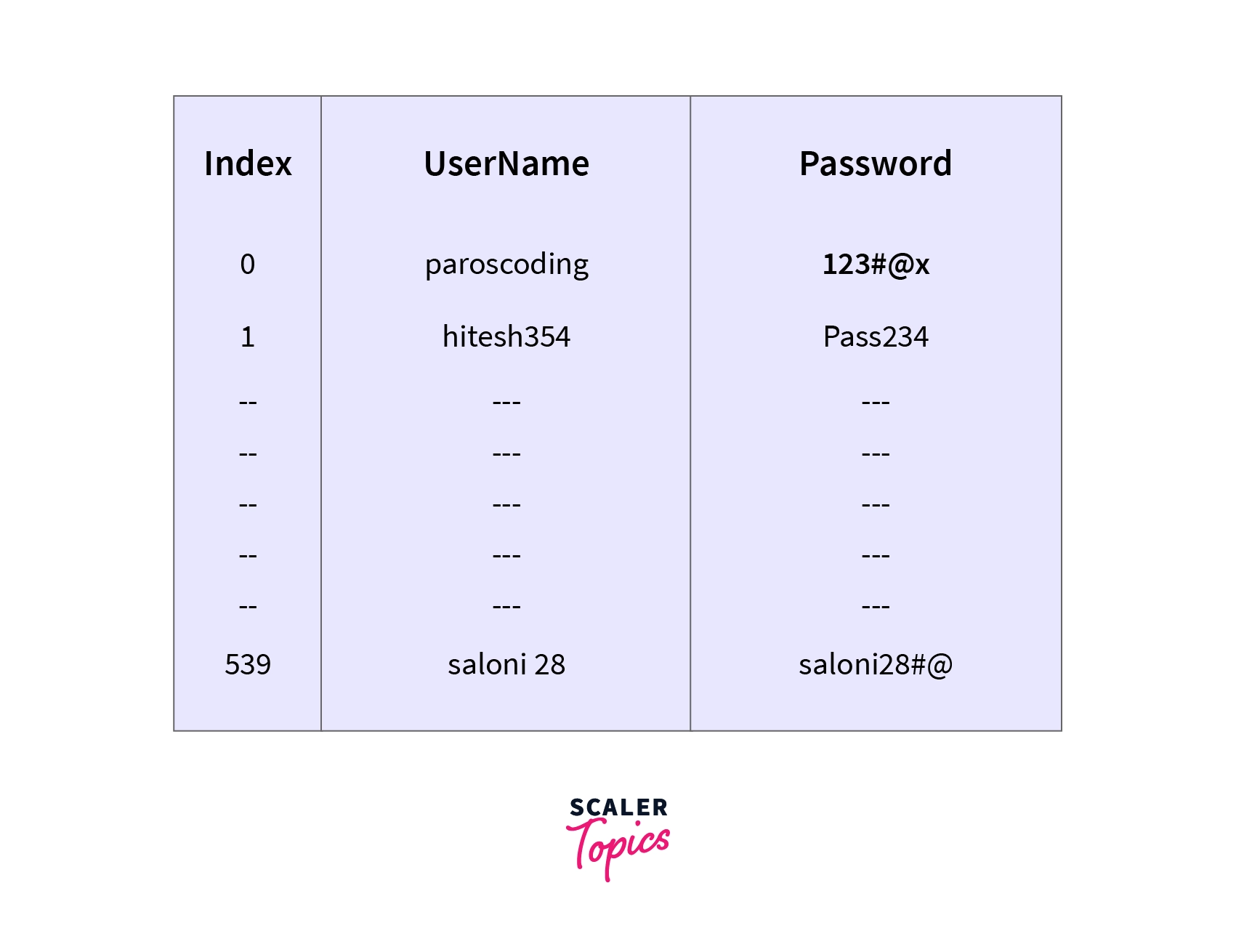The major drawbacks of this model are that it fails to store a huge amount of data secondly, to access any data whole table needs to be searched which makes it inefficient.

### Semi-Structured Data Model

A semi-structured data model is a generalized form of the relational model, which allows representing data in a flexible way, hence we can not differentiate between data and schema in this model because, in this model, some entities have a missing attribute(s) and on the other hand, some entities might have some extra attribute(s) which in turn makes it easy to update the schema of the database.
For example - We can say a data model is semi-structured if in some attributes we are storing both atomic values (values that can't be divided further, for example, Roll_No) as well as a collection of values.

### Associative Data Model

The associative data model sees the data in the same way as the brain does, $i.e.$ entities and relationships between them. The relationship is expressed as a simple English sentence of the form "subject-verb-object".

For example -
From the sentences

• Pulkit is a customer
• Pulkit's customer id is 645.
• Neeraj is a customer
• Neeraj's customer id is 784.

We can make the following table -

Cust_IDName
645Pulkit
784Neeraj

### Context Data Model

The context model is nothing but a combination of several data models that have been discussed above. For example, a context model can be a combination of a network model, ER model, etc. This data model allows one to do many things which were not possible if he/she use a single data model.

## Advantages of Data Models in DBMS

• Data models ensure that the data is represented accurately.
• The relationship between the data is well-defined.
• Data Redundancy in DBMS can be minimized and missing data can be identified easily.
• Last but not least, the security of the data is not compromised.

## Disadvantages of Data Models in DBMS

• The biggest disadvantage of the data model is, one must know the characteristics of physical data to build a data model.
• Sometimes in big databases, it is quite difficult to understand the data model also the cost incurred is very high.

## Conclusion

• Data models in DBMS are used to represent the logical structure of the database.
• Several types of data models exist with their own advantages and limitations.
• ER model, Relational model, and Object-oriented model are among the most popular data models in DBMS.